# Univariate, Bivariate and Multicollinearity Analysis in Machine Learning

## Univariate, Bivariate, and Multicollinearity Analysis in Machine Learning

In this article, I am going to discuss Univariate, Bivariate, and Multicollinearity Analysis in Machine Learning with Examples. Please read our previous article where we discussed Logistic Regression in Machine Learning with Examples.

##### Univariate Analysis in Machine Learning

Before diving deep into univariate analysis, let’s first understand what does it mean by univariate data?

There is only one variable in this type of data. Because the information only deals with one variable that varies, univariate data analysis is the simplest type of analysis. It is not concerned with causes or relationships, and the primary goal of the analysis is to describe the data and identify patterns.

Weight is an example of univariate data. Univariate analysis is used for the analysis of one single variable/feature. The goal of the univariate analysis is to derive data, characterize and summarize it, and examine any patterns that may exist. It investigates each variable separately in a dataset. There are two types of variables that can be used: categorical and numerical.

The central tendency measures (mean, median, and mode), data dispersion or spread (range, minimum, maximum, quartiles, variance, and standard deviation), and frequency distribution tables, histograms, pie charts, frequency polygons, and bar charts can all be used to describe patterns found in this type of data.

Let’s see the ways to analyze data on the weights of students –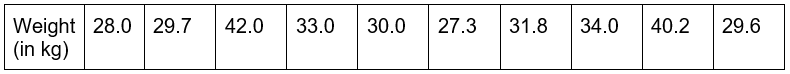##### Statistical Measures –

By using the df.describe() method, you can get all the statistical measures of a feature.

###### Example –
```import pandas as pd
import numpy as np
import matplotlib.pyplot as plt
import seaborn as sns

weights = {'weights':[28.0, 29.7, 42.0, 33.0, 30.0, 27.3, 31.8, 34.0, 40.2, 29.6]}

weights_df = pd.DataFrame(weights)
weights_df.describe()
```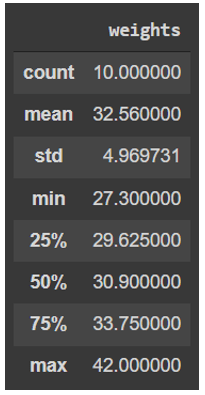##### Bar Charts –

When comparing categories of data or different groupings of data, the bar graph is extremely useful. It aids in the tracking of changes throughout time. It’s the ideal tool for displaying discrete data.

```sports = ['Football', 'Badminton', 'Chess']
enrollments = [43,32,25]
plt.bar(sports, enrollments)
plt.show()
```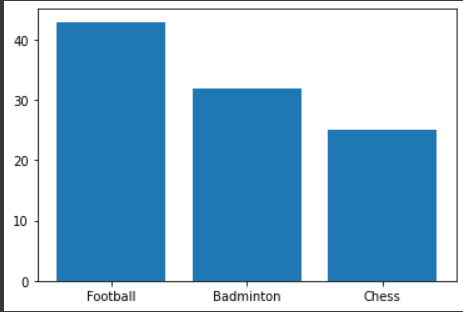##### Pie Charts –

Pie charts are commonly used to visualize how a group is divided into smaller parts. The entire pie symbolizes 100%, and the slices show the relative size of each group. Example –

```sports = ['Football', 'Badminton', 'Chess']
enrollments = [43,32,25]
plt.pie(enrollments, labels = sports)
plt.show()
```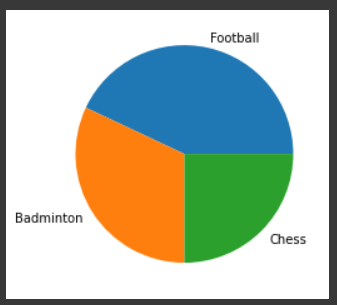##### Histograms –

Histograms are similar to bar charts in that they exhibit categorical variables against a specific data category. These categories are represented by bins in histograms, which reflect the number of data points in a range. It’s the greatest option for displaying continuous data. Example –

```a = np.array([23,22,19,17,19,25,24,23,22,26,28,22,21,20,25,23,25,23])
plt.hist(a)
plt.show()
```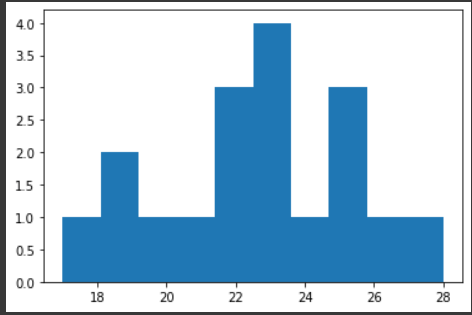#### Bivariate Analysis in Machine Learning

Before diving deep into univariate analysis, let’s first understand what does it mean by univariate data?

Two variables are involved in bivariate data. Bivariate analysis is concerned with causes and relationships, and the goal is to determine the relationship between the two variables. Example of bivariate data – temperature and ice cream sales in the summer.

Comparisons, correlations, causes, and explanations are all part of bivariate data analysis. One of these variables is independent, while the other is dependent, and these variables are frequently plotted on the X and Y axis on the graph for a better understanding of the data. Bivariate analysis can be divided into three categories –

#### Analysis of two Numerical Variables –

##### Scatter Plot –

A scatter plot uses dots to represent distinct pieces of data. These diagrams make it easy to see if two variables are connected. The pattern that results shows the kind (linear or nonlinear) and strength of the link between two variables.

###### Example –
```sales = {'Temperature':[38.0, 39.7, 42.0, 33.0, 30.0, 27.3, 31.8, 34.0, 40.2, 39.6], 'Sales':[37, 50, 55, 35, 31, 30, 34, 40, 50, 51]}
sales_df = pd.DataFrame(sales)

plt.scatter(sales_df['Temperature'], sales_df['Sales'])
plt.show()
```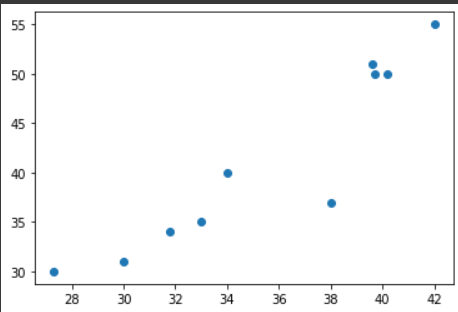##### Linear Correlation –

The strength of a linear link between two numerical variables is represented by linear correlation. The value of r denotes the strength of a linear relationship and is always between -1 and 1.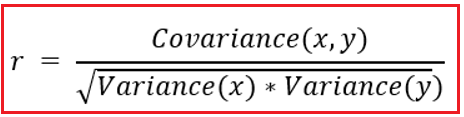The value of r equal to -1 signifies perfect negative linear correlation, +1 denotes perfect positive linear correlation, and zero denotes no linear correlation.

##### Analysis of two Categorical Variables –
###### Chi-Square Test

The chi-square test is used to determine whether category variables are related. The difference between expected and observed frequencies in one or more categories of the frequency table is used to calculate it. A probability of zero implies complete dependency between two categorical variables, while a probability of one indicates complete independence between two categorical variables.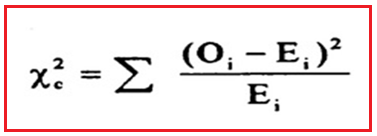The degrees of freedom are denoted by the subscript c, the observed value is O, and the predicted value is E.

##### Analysis of One Numerical and One Categorical Variable –
###### Z-Test and T-Test –

Z and T tests are used to determine if there is a significant difference between a sample and the population. The difference between the two averages is more noticeable when the likelihood of Z is small.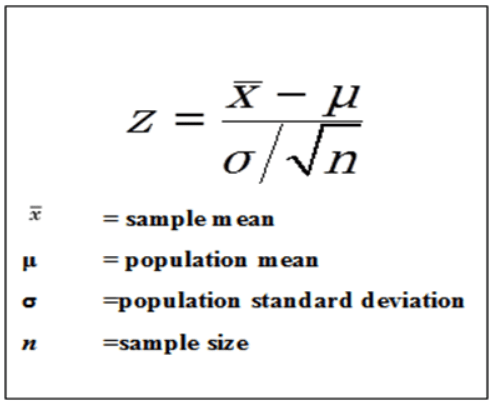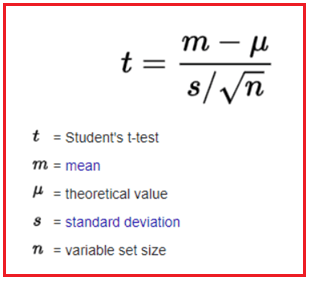We employ a Z-test if the sample size is large enough, and a T-test if the sample size is tiny.

##### Multicollinearity Analysis in Machine Learning

Multicollinearity (also known as collinearity) is a statistical phenomenon in which one feature variable in a regression model has a high linear correlation with another feature variable. When two or more variables are perfectly correlated, this is referred to as collinearity.

When independent variables are highly linked, a change in one causes a change in the others, causing the model results to fluctuate a lot. Given a slight change in the data or model, the model outcomes will be unstable and fluctuate a lot. The following issues will arise as a result of multicollinearity:

1. If the model provides you with different results every time, it will be difficult for you to determine the list of significant variables for the model.
2. Coefficient Estimates would be unstable, making it difficult to interpret the model. In other words, if one of your predictive factors changes by one unit, you can’t determine how much the output will change.
3. Overfitting may occur due to the model’s instability. When you apply the model to a different set of data, the accuracy will be much lower than it was with your training dataset.

If just minor or moderate collinearity occurs, it may not be a problem for your model, depending on the situation. However, if there is a serious collinearity problem (e.g., a correlation of >0.8 between two variables), it is strongly encouraged to tackle the problem.

##### How to check multicollinearity?
###### Correlation Matrix –

The correlation matrix of all independent variables is plotted as the first basic technique. We can see the pairwise correlation between all the variables after plotting the correlation matrix and color scaling the background. Example –

```df = sns.load_dataset('mpg')

#plot color scaled correlation matrix
corr=df.corr()
```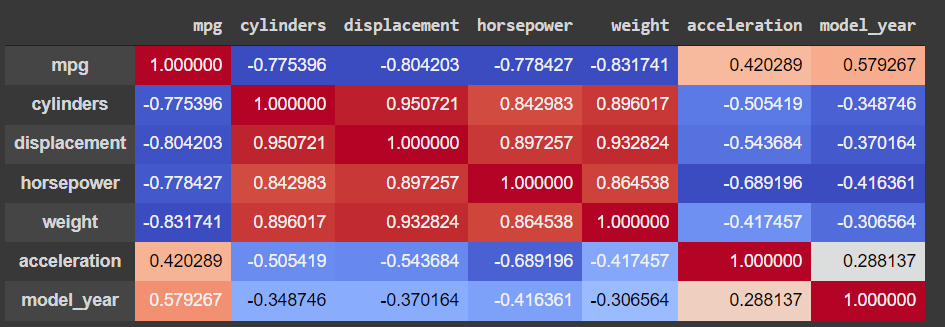Here, we can see that many independent features are highly correlated.

##### The Variance Inflation Factor (VIF) –

VIF for each independent variable is the second method for determining multicollinearity. It’s a metric for determining how multicollinear a set of multivariate regression variables is. The higher the VIF value, the stronger the link between one variable and the others.

If the VIF number is greater than 10, it is usually assumed that the independent variables are highly correlated. The acceptable range, on the other hand, is limited by requirements and limits. Example –

```#Let's check VIF data for each independent variable
from statsmodels.stats.outliers_influence import variance_inflation_factor
vif = pd.DataFrame()
vif["features"] = df.columns
vif["vif_Factor"] = [variance_inflation_factor(df.values, i) for i in range(df.shape)]
vif
```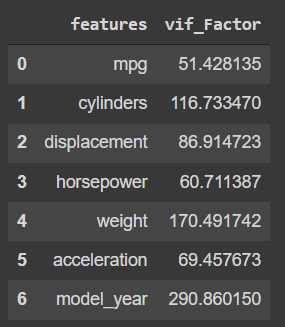In the next article, I am going to discuss Model Building and Validation in Machine Learning with Examples. Here, in this article, I try to explain Univariate, Bivariate, and Multicollinearity Analysis in Machine Learning with Examples. I hope you enjoy this Univariate, Bivariate, and Multicollinearity Analysis in Machine Learning with Examples article.